#Quality Digest

Featured Product
This Week in Quality Digest Live
Six Sigma Features
Anthony D. Burns
Upcoming interactive mobile app demonstrates Deming’s process variation experiment
Tom Taormina
Champion business success and avoid risk
Michael Popenas
Enables companies to develop, design, test, and release products and services more quickly than other waterfall methods
James J. Kline
A sound quality management foundation exists in local government
Greg Hutchins
Risk is becoming our lens for everything from managing, to working, and even going to the store.
Six Sigma News
Too often process enhancements occur in silos where there is little positive impact on the big picture
Collect measurements, visual defect information, simple Go/No-Go situations from any online device
Good quality is adding an average of 11 percent to organizations’ revenue growth
Floor symbols and decals create a SMART floor environment, adding visual organization to any environment
A guide for practitioners and managers
Making lean Six Sigma easier and adaptable to current workplaces
Gain visibility into real-time quality data to improve manufacturing process efficiency, quality, and profits
Makes it faster and easier to find and return tools to their proper places
Version 3.1 increases flexibility and ease of use with expanded data formatting featuresBio

Six Sigma

## Problems with Skewness and Kurtosis, Part One

### What do the shape parameters do?

Published: Friday, July 29, 2011 - 15:30

With the use of statistical software, many individuals are being exposed to more than just measures of location and dispersion. In addition to the average and standard deviation, they often find some funny numbers labeled as skewness and kurtosis. Since these numbers appear automatically, it is natural to wonder how they might be used in practice. In part one of this two-part column, I'll illustrate what the skewness and kurtosis parameters do. In part two I will look at the use of skewness and kurtosis statistics provided by software packages.

Since the previous sentence makes a distinction between a statistic and a parameter, we should begin there. Statistics are merely functions of the data. We find the value for a statistic by performing a set of arithmetic operations using a set of data. For example, we compute the average for a set of numbers by adding up all the numbers and dividing by the number of values in the sum. Thus, any time we have a collection of numbers we can compute any one of a number of statistics. Data plus arithmetic equals a statistic.

On the other hand, a parameter is a descriptive constant for a probability model. Parameters are used to characterize specific properties of a probability model. This means that, rather than using data, parameters are obtained by performing certain mathematical operations using the probability model. Since probability models must meet certain requirements, parameters are not well defined until the probability model is well defined.

The first four parameters for a probability model are the mean, the variance, the skewness, and the kurtosis. Given a continuous probability model characterized by the probability density function f(x), the mean of the probability model will characterize the location and is defined as: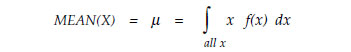The variance of the probability model will characterize the dispersion and is defined as:The square root of the variance is commonly known as the standard deviation. It provides an alternative way to characterize the dispersion of the probability model: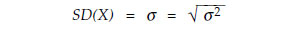The skewness and kurtosis are collectively known as the shape parameters for the probability model. The skewness parameter for the probability model is defined to be the third standardized central moment. This means that we begin with the standardized form for the random variable: [(x – μ)/σ ], raise it to the third power, multiply by the probability model, and integrate over all x.In a similar manner, the kurtosis parameter for the probability model is defined as the fourth standardized central moment:At this point it should be abundantly clear why you never computed the skewness and kurtosis parameters in your stat class. Moreover, since you do not routinely evaluate integrals, it is fairly safe to say that you have probably not computed any parameters since you finished (or dropped out of) your stat class. However, because these parameters characterize various aspects of a probability model, they are useful in organizing the zoo of probability models.

To illustrate how the skewness and kurtosis parameters characterize the shape of a probability model, we shall use a simple probability model for which the integrals above will be easy to illustrate and evaluate. This probability model is the standardized right triangular distribution. It has a probability density function f(x) of: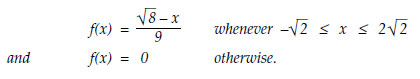This probability model has a mean of zero, a standard deviation of 1.000, and is shown in
figure 1.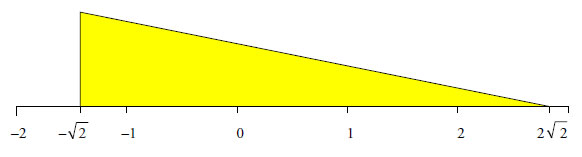Figure 1: The Standardized Right Triangular Distribution

Since this is a standardized distribution, the standardized form for the random variable reduces down to simply [x]. Thus, the formulas for the skewness and kurtosis parameters reduce to the following: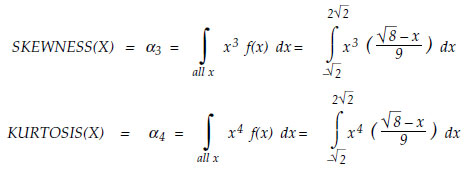Thus, we see that in this case, the skewness is the integral of the product of the cubic curve and the density function, while the kurtosis is the integral of the product between the quartic curve and the density function. Figure 2 shows the density function along with the cubic and quartic curves. Figures 3 and 4 show the resulting product curves.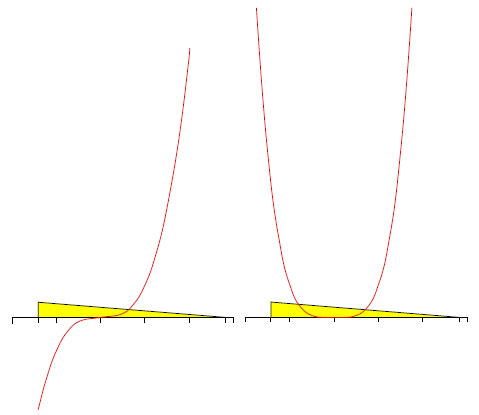Figure 2: Cubic and Quartic Curves with f(x)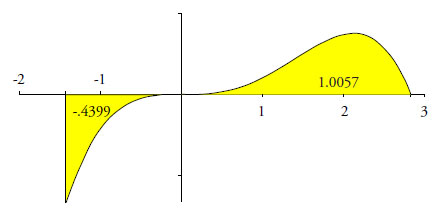Figure 3: The Areas That Define the Skewness Parameter

Interpreting the integral as the area between the product curve and the X axis, we find that the skewness parameter for this probability model may be interpreted as:Figure 4 shows the curve that results when we multiply the probability model by the quartic curve. The kurtosis parameter for this probability model may be interpreted as the area under the curve in figure 4. In this case: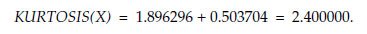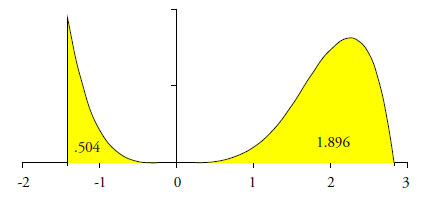Figure 4: The Areas That Define the Kurtosis Parameter

The fact that all four regions in figures 3 and 4 pinch down near zero suggests that the central region of the probability model contributes very little to either of these two parameters. Since the distribution in this example is already in its standardized form, the units on the horizontal axis in figures 3 and 4 represent the standardized distance from the mean. Thus, the contribution of the central portion of the probability model can be seen by considering how much of the total area under the curves corresponds to X values which fall between –1.0 and +1.0.

While the central portion of this probability model contributes 63 percent of the total area, only 11 percent of the combined areas in figure 3, and only 5 percent of the area in figure 4, correspond to the central portion of the probability model. Therefore, we must conclude that both skewness and kurtosis are primarily concerned with characteristics of the tails of the probability model.Figure 5: Skewness and Kurtosis Characterize the Tails of a Probability Model

The skewness parameter measures the relative sizes of the two tails. Distributions that have tails of equal weight will have a skewness parameter of zero. If the right-hand tail is more massive, then the skewness parameter will be positive. If the left-hand tail is more massive, the skewness parameter will be negative. Moreover, the greater the difference between the two tails, the greater the magnitude of the skewness parameter.

The kurtosis parameter is a measure of the combined weight of the tails relative to the rest of the distribution. As the tails of a distribution become heavier, the kurtosis will increase. As the tails become lighter, the kurtosis will decrease. As defined here kurtosis cannot be less than 1.00. Probability models with kurtosis values between 1.00 and 3.00 are considered to be light-tailed distributions (platykurtic). Probability models with kurtosis values in excess of 3.00 are considered to be heavy-tailed distributions (leptokurtic).

Kurtosis was originally thought to measure the "peakedness" of a distribution. However, since the central portion of the distribution is virtually ignored by this parameter, kurtosis cannot be said to measure peakedness directly. While there is a correlation between peakedness and kurtosis, the relationship is an indirect and imperfect one at best.

Thus, the shape parameters of skewness and kurtosis actually tell us more about the tails of a probability model than they do about the central portion of that model. At the beginning of the 20th century the shape parameters were used simply because Karl Pearson had developed seven families of probability models that were fully characterized by the first four moments. Of these families the two most important are the Beta Distributions (Pearson Type One) and the Gamma Distributions (Pearson Type Three).

By plotting the values of the shape parameters on Cartesian coordinates, Pearson was able to show how these families of probability models were related to each other. This plot is known as the shape characterization plane. In this plane a probability model is represented by a single point, while families of probability models will sometimes fall on a line or fall within in a region of the plane. For example, all normal distributions will have a skewness of zero and a kurtosis of 3.00. In the shape characterization plane, the skewness squared defines the X-coordinate, while the kurtosis defines the Y-coordinate. Thus, the family of all normal distributions will be shown on the shape characterization plane by a single point at (0, 3). The family of all exponential distributions (skewness = 2, kurtosis = 9) will be shown by a single point at (4, 9).Figure 6: The Beta and Gamma Families of Distributions on the Shape Characterization Plane

Figure 6 shows the heart of the shape characterization plane. The gamma distributions are represented by the line defined by the normal and exponential distributions. All of the chi-square distributions fall on this line. The beta distributions occupy the whole region of the plane below the gamma distribution line. The shape characterization plane can be divided as shown into regions according to whether the probability models are mound-shaped, J-shaped, or bimodal. At the apex of the dividing lines between these three divisions, we find the family of uniform distributions, which are neither mound-shaped, J-shaped, nor bimodal.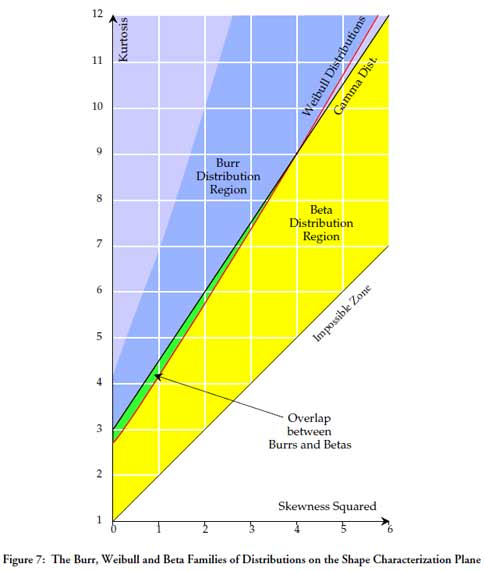Figure 7: The Burr, Weibull, and Beta Families of Distributions on the Shape Characterization Plane

Figure 7 shows the family of positively skewed Weibull distributions as a red line. Above this line we find the family of Burr distributions effectively covering the rest of the region of mound-shaped probability models. Thus, skewness and kurtosis parameters are useful because of their ability to characterize and organize the zoo of probability models. Moreover, as seen in figures 6 and 7, the families of the betas and Burrs, plus their limiting families of the gammas and the Weibulls will effectively cover the whole shape characterization plane. Does this mean that these are the only probability models? By no means. But it does mean that a first order approximation to virtually any probability model can be found among these four families of distributions.

The reason that these distributions will only provide a first order approximation is due to the fact that the skewness and kurtosis only characterize the tails of the distribution. This is why it is fallacious to think that two distributions having the same mean, standard deviation, skewness, and kurtosis will have exactly the same shape. A second, related fallacy is that a distribution with a skewness parameter of zero will be symmetric. That these are indeed fallacies will be illustrated by the following examples.

Figure 8 shows a simple symmetric probability model characterized by the density function, f(x) where: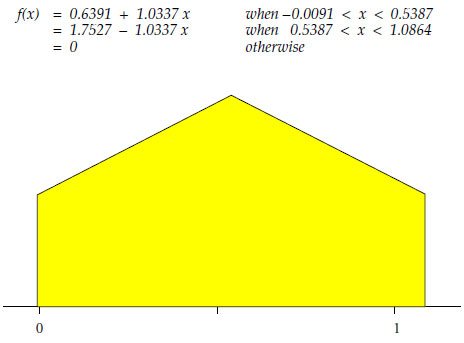Figure 8: A Simple Symmetric Probability Density Function

The probability model in figure 8 has a mean of 0.5387, a standard deviation of 0.2907, a skewness of 0.000, and a kurtosis of 2.000. The symmetry of this distribution requires a skewness of zero, and the short tails result in a small value for kurtosis.Figure 9: The Elephant Distribution

Figure 9 shows a nonsymmetric probability model from the family of Inverse Burr distributions, which are characterized by the density function g(x):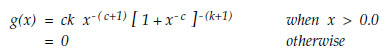When we let the value of c be 18.1484, and let the value of k be 0.0629, we get the probability model shown in figure 9. This probability model has a mean of 0.5387, a standard deviation of 0.2907, a skewness of 0.0000, and a kurtosis of 2.0000. With a couple of extra lines, this distribution can be made into a reasonable cartoon of an elephant. While this probability model is definitely not symmetric, it does have a skewness of zero. Moreover, like the probability model in figure 8, it also has a kurtosis of 2.00.

Figure 10 compares the distributions of figures 8 and 9. There we find two distributions that have the same mean, the same standard deviation, the same skewness, and the same kurtosis, yet they do not look alike. Thus, we may properly conclude that the "shape parameters" of skewness and kurtosis cannot even discriminate between an elephant and the gable end of a house!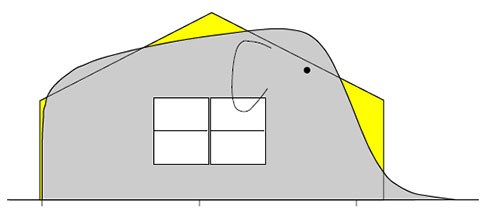Figure 10: Two Probability Models with the Same Mean, Variance, and Shape Parameters

Although probability models having the same shape parameters will display a gross similarity, they do not have to be exactly alike. While most of these differences might be expected to occur in the central portion of the distributions, as we can see in figure 8, some of these differences can also occur in the tails.

This column has focused on the shape parameters for probability models. In part two we will consider the utility of shape statistics. However, before getting lost in this world of probability models, it is important to note that we cannot begin to use a probability model to approximate reality until we have a predictable process. Any attempt to choose, fit, or otherwise use a probability model to characterize an unpredictable process is a mistake.

### Discuss